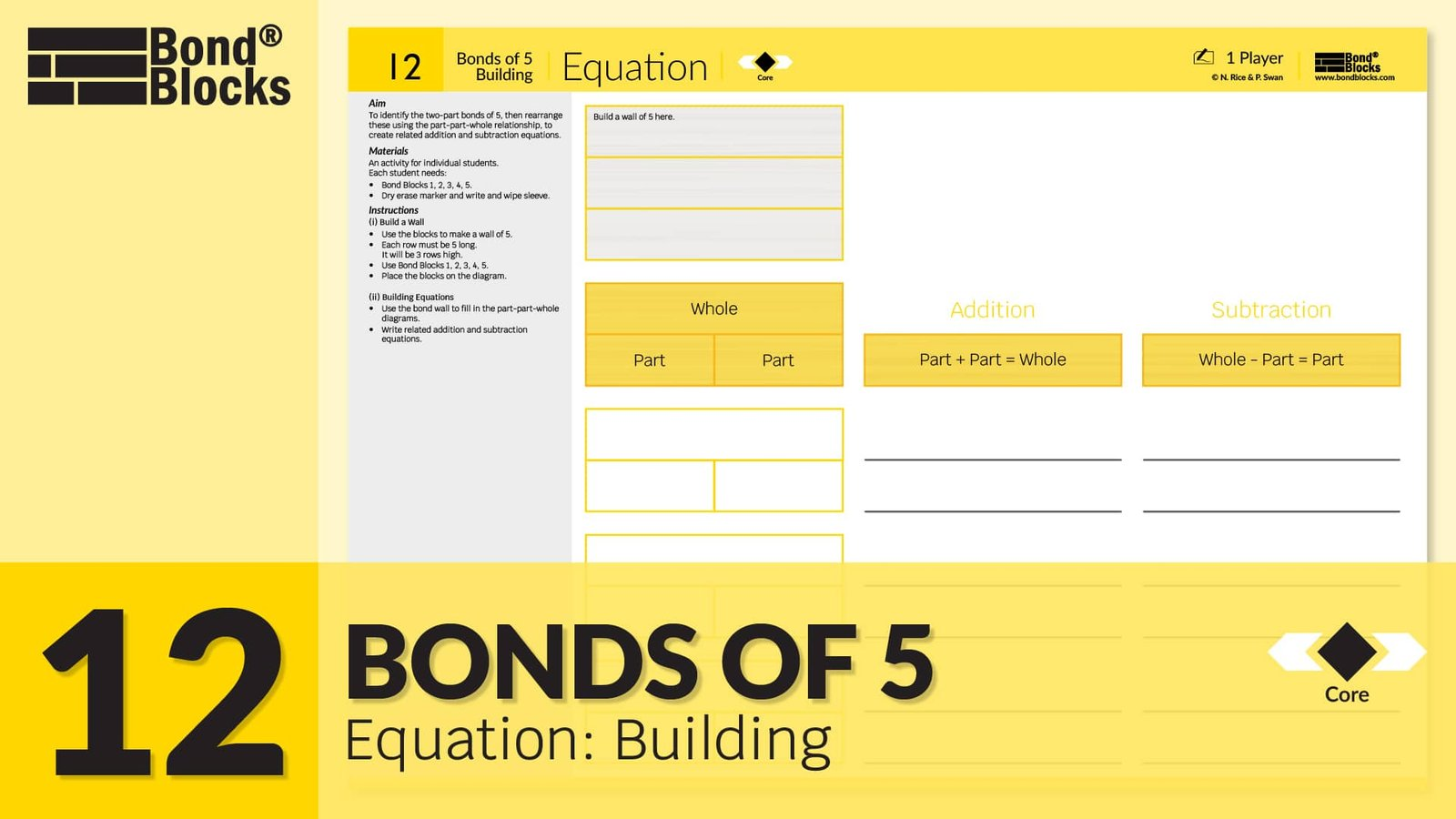## 12) Equation

Bonds of 5: Building### Mathematics

Develop the concept of related addition and subtraction equations, rearranged using the part-part-whole relationship, for two-part bonds of 5.

### Language

• part-part-whole: where the two parts are a bond of the whole number.
• equals, is equal to
• subtraction, subtract, take away

### Core Activity Support MaterialsClick to open in a new tab.## Differentiation

### A little easier

##### Equation building

Use Bonds of 5: Equation Building a little easier activity board to scaffold building addition and subtraction equations for one bond of 5.

• Arrange each block, in the correct positions, on the rectangles of the first equation.
• Record these numbers using a dry erase marker.
• Repeat for each equation.

At the end of the activity discuss:

• The different position of the whole in addition equations compared to subtraction.
• How the blocks representing the parts swap positions with each other. The block representing the whole stays in the same position for each addition equation and each subtraction equation.### Progression

Apply the concept of building related addition and subtraction equations, using part-part-whole, to find missing parts in addition and subtraction equations. Go to

##### Activity 13

Bonds of 5: Missing Number Equations, Fill a Row